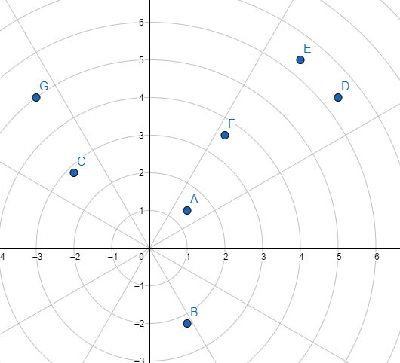# Program to sort given set of Cartesian points based on polar angles in Python

Suppose we have a set of Cartesian points in a list called points. We have to sort them based on their polar angles. The polar angles vary in range 0 and 2*PI. If some points have same polar angles, then arrange them based on the distance of that point from the origin.

So, if the input is like points = [(1,1), (1,-2),(-2,2),(5,4),(4,5),(2,3),(-3,4)],then the output will be [(5, 4), (1, 1), (4, 5), (2, 3), (-3, 4), (-2, 2), (1, -2)]To solve this, we will follow these steps −

• Define a comparator function key() . This will take x
• atan := tan-inverse of x/x
• return pair (atan, x^2+x^2) if atan >= 0 otherwise (2*pi + atan, x^2+x^2)
• then sort points using comparator function key()

## Example

Let us see the following implementation to get better understanding −

import math
def solve(points):
def key(x):
atan = math.atan2(x, x)
return (atan, x**2+x**2) if atan >= 0 else (2*math.pi + atan, x**2+x**2)

return sorted(points, key=key)

points = [(1,1), (1,-2),(-2,2),(5,4),(4,5),(2,3),(-3,4)]
print(solve(points))

## Input

[(1,1), (1,-2),(-2,2),(5,4),(4,5),(2,3),(-3,4)]


## Output

[(5, 4), (1, 1), (4, 5), (2, 3), (-3, 4), (-2, 2), (1, -2)]